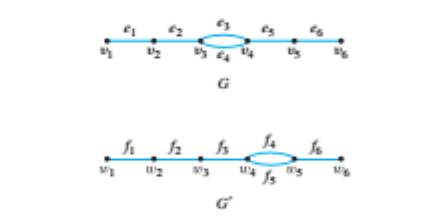Chapter 10.3, Problem 30ES### Discrete Mathematics With Applicat...

5th Edition
EPP + 1 other
ISBN: 9781337694193

#### Solutions

Chapter
Section### Discrete Mathematics With Applicat...

5th Edition
EPP + 1 other
ISBN: 9781337694193
Textbook Problem
1 views

# Show that the following two graphs are not isomorphic by supposing they are isomorphic and deriving a contradiction.To determine

To prove:

Show that the following two graphs are not isomorphic by supposing they are isomorphic and deriving a contradiction.

Explanation

Given information:

Proof:

Let us assume that G and G are isomorphic. We then need to derive a contradiction.

Let V ( G ) be the set of vertices of G and let V(G) be the set of vertices of G.

V(G)={v1,v2,v3,v4,v5,v6}V(G)={w1,w2,w3,w4,w5,w6}

Let E (G) be the set of edges of G and let E(G) be the set of edges of G.

E(G)={e1,e2,e3,e4,e5,e6}E(G)

### Still sussing out bartleby?

Check out a sample textbook solution.

See a sample solution

#### The Solution to Your Study Problems

Bartleby provides explanations to thousands of textbook problems written by our experts, many with advanced degrees!

Get Started